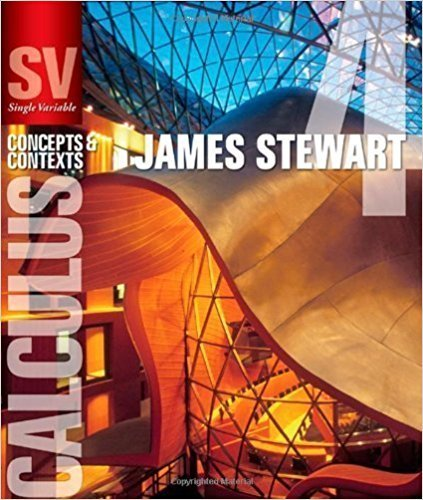×
×

# Solutions for Chapter 6.2: VOLUMES## Full solutions for Single Variable Calculus: Concepts and Contexts (Stewart's Calculus Series) | 4th Edition

ISBN: 9780495559726Solutions for Chapter 6.2: VOLUMES

Solutions for Chapter 6.2
4 5 0 261 Reviews
29
5
##### ISBN: 9780495559726

This expansive textbook survival guide covers the following chapters and their solutions. This textbook survival guide was created for the textbook: Single Variable Calculus: Concepts and Contexts (Stewart's Calculus Series), edition: 4. Single Variable Calculus: Concepts and Contexts (Stewart's Calculus Series) was written by and is associated to the ISBN: 9780495559726. Since 54 problems in chapter 6.2: VOLUMES have been answered, more than 21917 students have viewed full step-by-step solutions from this chapter. Chapter 6.2: VOLUMES includes 54 full step-by-step solutions.

Key Calculus Terms and definitions covered in this textbook
• Additive identity for the complex numbers

0 + 0i is the complex number zero

• Arcsine function

See Inverse sine function.

• Binomial

A polynomial with exactly two terms

• Completing the square

A method of adding a constant to an expression in order to form a perfect square

• Composition of functions

(f ? g) (x) = f (g(x))

• Distance (in Cartesian space)

The distance d(P, Q) between and P(x, y, z) and Q(x, y, z) or d(P, Q) ((x ) - x 2)2 + (y1 - y2)2 + (z 1 - z 2)2

• Equally likely outcomes

Outcomes of an experiment that have the same probability of occurring.

• Head minus tail (HMT) rule

An arrow with initial point (x1, y1 ) and terminal point (x2, y2) represents the vector <8x 2 - x 1, y2 - y19>

• Integers

The numbers . . ., -3, -2, -1, 0,1,2,...2

• Interquartile range

The difference between the third quartile and the first quartile.

• Negative linear correlation

See Linear correlation.

• One-to-one function

A function in which each element of the range corresponds to exactly one element in the domain

• Paraboloid of revolution

A surface generated by rotating a parabola about its line of symmetry.

• Positive angle

Angle generated by a counterclockwise rotation.

• Solve a system

To find all solutions of a system.

• Speed

The magnitude of the velocity vector, given by distance/time.

• Tangent

The function y = tan x

• Terminal point

See Arrow.

• Unit vector

Vector of length 1.

• Variation

See Power function.

×